Solution of self-consistent equations for the NLO nuclear energy density functional in spherical symmetry. The program HOSPHE (v1.00)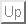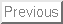Next: Introduction Up: Jacek Dobaczewski - strona domowa

# Solution of self-consistent equations for the N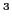LO nuclear energy density functional in spherical symmetry. The program HOSPHE (v1.00)

B.G. Carlsson, J. Dobaczewski, J. Toivanen, P. Veselý

### Abstract:

We present solution of self-consistent equations for the NLO nuclear energy density functional. We derive general expressions for the mean fields expressed as differential operators depending on densities and for the densities expressed in terms of derivatives of wave functions. These expressions are then specified to the case of spherical symmetry. We also present the computer program HOSPHE (v1.00), which solves the self-consistent equations by using the expansion of single-particle wave functions on the spherical harmonic oscillator basis.

PROGRAM SUMMARY

Manuscript Title: Solution of self-consistent equations for the NLO nuclear energy density functional in spherical symmetry.
Authors: B.G. Carlsson, J. Dobaczewski, J. Toivanen, and P. Veselý
Program Title: HOSPHE (v1.00)
Journal Reference:
Catalogue identifier:
Licensing provisions: none
Programming language: FORTRAN-90
Operating system: Linux
RAM: 50MB
Number of processors used: 1
Keywords: Hartree-Fock, Skyrme interaction, nuclear energy density functional, self-consistent mean-field
PACS: 07.05.Tp, 21.60.-n, 21.60.Jz
Classification: 17.22 Hartree-Fock Calculations
External routines/libraries: LAPACK, BLAS
Nature of problem:
The nuclear mean-field methods constitute principal tools of a description of nuclear states in heavy nuclei. Within the Local Density Approximation with gradient corrections up to NLO , the nuclear mean-field is local and contains derivative operators up to sixth order. The locality allows for an effective and fast solution of the self-consistent equations.
Solution method:
The program uses the spherical harmonic oscillator basis to expand single-particle wave functions of neutrons and protons for the nuclear state being described by the NLO nuclear energy density functional . The expansion coefficients are determined by the iterative diagonalization of the mean-field Hamiltonian, which depends non-linearly on the local neutron and proton densities.
Restrictions:
Solutions are limited to spherical symmetry. The expansion on the harmonic-oscillator basis does not allow for a precise description of asymptotic properties of wave functions.
Running time:
50 sec. of CPU time for the ground-state of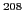Pb described by using the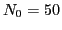maximum harmonic-oscillator shell included in the basis.
References:


B.G. Carlsson, J. Dobaczewski, and M. Kortelainen, Phys. Rev. C 78, 044326 (2008).

LONG WRITE-UPNext: Introduction
Jacek Dobaczewski 2010-01-30